# 记一次手撕算法面试：字节跳动的面试官把我四连击了

#### 一、小牛试刀：有效括号

示例 1:

实例 2：
输入: "())("



1、在遍历字符串的过程中，遇到 “(” 就让它入栈，遇到 “)” 就判断下栈里面有没有 “(” ：

（1）、如果有，则把处于栈顶的 "("  弹出，相当于和 ")" 进行匹配，然后继续往后遍历字符串

（2）、如果没有，则匹配失败。相当于字符串的最前面出现了 ")"，显然这是不合理的。


2、当字符串遍历完成，判断栈是否为空，如果为空则表示字符串有效，否则无效。public static boolean isValid(String s){
if(s == null || s.length() < 1)
return true;
int n = s.length();// 字符串长度
// 创建一个栈来装字符
Stack<Character> stack = new Stack<>();
// 遍历字符串
for(int i = 0; i < n; i++){
// 获取字符串的第 i 个字符
char c = s.charAt(i);
if(c == '('){
stack.push(c);
}else{
if(stack.isEmpty())
return false;
else
stack.pop();
}
}
// 判断是否为空
if(stack.isEmpty())
return true;

return false;
}


#### 二、优化

public static boolean isValid(String s){
if(s == null || s.length() < 1)
return true;
int n = s.length();// 字符串长度
// 用来记录遇到的 "(" 的个数
int sum = 0;
// 遍历字符串
for(int i = 0; i < n; i++){
// 获取字符串的第 i 个字符
char c = s.charAt(i);
if(c == '('){
sum++;
}else{
if(sum == 0)
return false;
else
sum--;
}
}
return sum == 0 ? true : false;
}


#### 三、最长有效括号

示例 1:



1、暴力法

…..

2、优化

1、先把 -1 放入栈内。（至于为什么？看到后面你就知道了）

2、、对于遇到的每个 ‘(‘ ，我们将它的下标放入栈中。

3、对于遇到的每个 ‘)’ ，我们弹出栈顶的元素并将当前元素的下标与遇到的‘(’元素的下标作差，得出当前有效括号字符串的长度

0、初始化：max = 0; i = 0。-1 放入栈内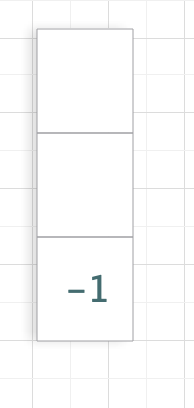1、i = 0，s[i] = ‘(‘，下标 i = 0 入栈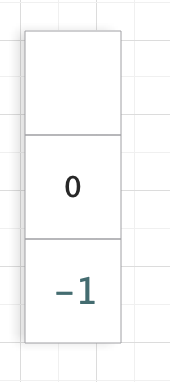2、i = 1，s[i] = ‘)’，出栈; i – 栈顶元素 = 1 – (-1) = 2，此时 max = 2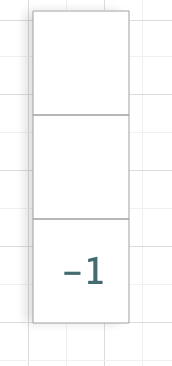3、i = 2，s[i] = ‘)’，出栈；这个时候要注意：由于 -1 出栈后，栈顶没有元素了，所以这个时候我们必须把 ‘)’ 的下标入栈，相当于最开始的初始化。4、i = 3，s[i] = ‘(‘，入栈;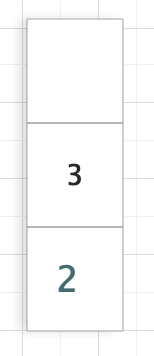5、i = 4，s[i] = ‘(‘，入栈;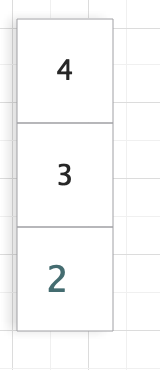6、i = 5，s[i] = ‘)’，出栈；i – 栈顶 = 5 – 3 = 2；此时 max = 2;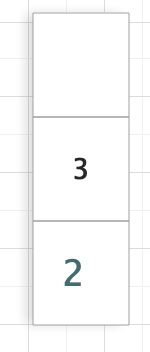7、i = 6，s[i] = ‘)’，出栈；i – 栈顶 = 6 – 2 = 4；此时 max = 4;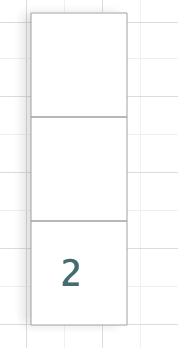8、遍历结束，最长有效括号为 4。

public int longestValidParentheses(String s) {
int max = 0;
Stack<Integer> stack = new Stack<>();
stack.push(-1);
for (int i = 0; i < s.length(); i++) {
if (s.charAt(i) == '(') {
//下标入栈
stack.push(i);
} else {
// 出栈
stack.pop();
// 看栈顶是否为空，为空的话就不能作差了
if (stack.empty()) {
stack.push(i);
} else {
// i - 栈顶，获得档期有效括号长度
max = Math.max(max, i - stack.peek());
}
}
}
return maxans;
}


#### 4、最后一击

1、如果 left right，显然这个时候 right 个 ‘)’ 都将一定能够得到匹配。所以当前的有效括号长度为 2 * right。然后更新 max。

2、如果 left < right，显然这个时候部分 ‘)’ 一定得不到匹配，此时我们把 left 和 right 都置为 0。

public int longestValidParentheses(String s) {
if(s == null || s.length() < 1)
return 0;
int left = 0, right = 0, max = 0;
// 从左到右
for (int i = 0; i < s.length(); i++) {
if (s.charAt(i) == '(') {
left++;
} else {
right++;
}
if (left == right) {
max = Math.max(max, 2 * right);
} else if(right > left){
left = right = 0;
}
}
left = right = 0;
// 从右到左
for (int i = s.length() - 1; i >= 0; i--) {
if (s.charAt(i) == '(') {
left++;
} else {
right++;
}
if (left == right) {
max = Math.max(max, 2 * left);
} else if (left > right) {
left = right = 0;
}
}
return max;
}